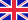•# Random Vectors and Random Sequences

Autor: Robert Piché

This textbook covers the essential theory of random vectors and sequences, an understanding of which is essential for understanding and applying estimation, filtering and tracking algorithms. Requiring only a knowledge of basic probability and of matrix... Viac o knihe

Na objednávku, dodanie 2-4 týždne

59.50 €

bežná cena: 70.00 €

## O knihe

This textbook covers the essential theory of random vectors and sequences, an understanding of which is essential for understanding and applying estimation, filtering and tracking algorithms. Requiring only a knowledge of basic probability and of matrix algebra, it begins with a succinct review of probability theory, starting with univariate discrete variables and proceeding step by step to the continuous multivariate case. It then presents multivariate normal (Gaussian) estimation of linear model parameters, random sequences (including convergence, ergodicity, and power spectral density), state space models of linear discrete-time dynamic systems and their response to random inputs, and finally the Kalman filter algorithm. Distinguishing features of this textbook include full coverage of multivariate theory, a modern (Bayesian) approach to estimation and filtering, detailed proofs of key results, and 75 exercises with answers.

• Vydavateľstvo: LAP LAMBERT Academic Publishing
• Rok vydania: 2012
• Formát: Paperback
• Rozmer: 220 x 150 mm
• Jazyk: Anglický jazyk
• ISBN: 9783659211966

Generuje redakčný systém BUXUS CMS spoločnosti ui42.